Premium

# 5.NF.B.6 Worksheets, Workbooks, Lesson Plans, and Games

#### CCSS.maths.CONTENT.5.NF.B.6

:
"Solve real world problems involving multiplication of fractions and mixed numbers, e.g., by using visual fraction models or equations to represent the problem."

These worksheets and lesson plans can help students practise this Common Core State Standards skill.

## Worksheets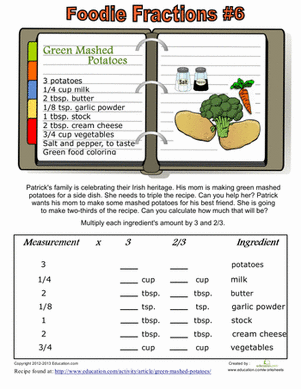Recipe Fractions #6
Worksheet
Recipe Fractions #6
Fraction practise can be fun when you add food into the mix! Your child will multiply fractions to change the amounts of ingredients in a recipe.
Year 6
Maths
Worksheet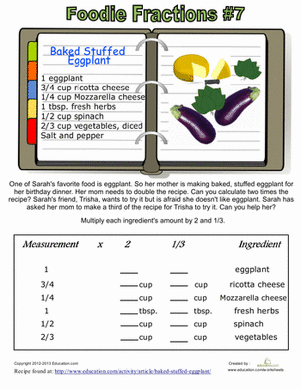Recipe Fractions #7
Worksheet
Recipe Fractions #7
Practise multiplying fractions using a real recipe. Your child will have a chance to learn about food as she learns about maths.
Year 6
Maths
Worksheet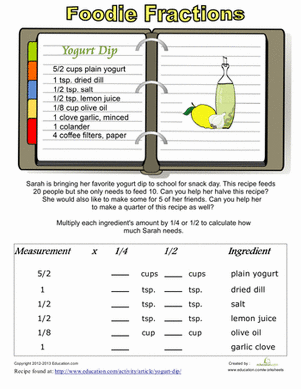Recipe Conversion
Worksheet
Recipe Conversion
Introduce your little maths champ to fraction multiplication in the kitchen! He'll get a taste of measurements using a recipe to calculate the fractions.
Year 6
Maths
Worksheet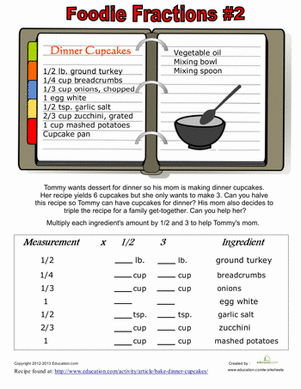Recipe Fractions #2
Worksheet
Recipe Fractions #2
Use food and maths together to learn fractions! Help your child multiply fractions to convert these recipes to smaller or larger portions.
Year 6
Maths
Worksheet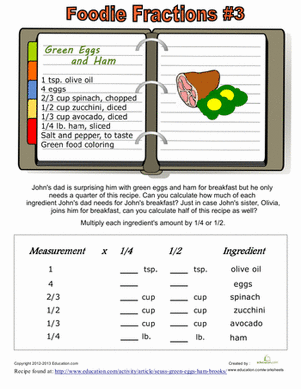Recipe Fractions #3
Worksheet
Recipe Fractions #3
Combine maths with the fun of cooking! practise fraction multiplication using the measurements of ingredients from a recipe.
Year 6
Maths
Worksheet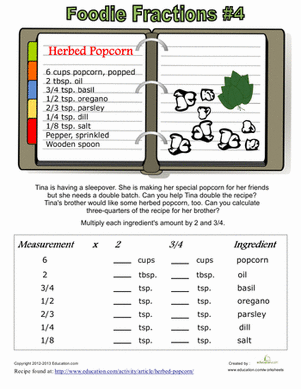Recipe Fractions #4
Worksheet
Recipe Fractions #4
Practise multiplying fractions while learning to cook in the kitchen! Your little mathematical chef will adjust the measurements of a real recipe.
Year 6
Maths
Worksheet

## Lesson PlansMixed Word Problems with RDW Strategy
Lesson plan
Mixed Word Problems with RDW Strategy
This end-of-the-school-year lesson will get the students focused on all the things they learned in maths class! It reviews different skills with fractions and decimals in word problems using the "Read, Draw, and Write" strategy.
Year 6
Maths
Lesson plan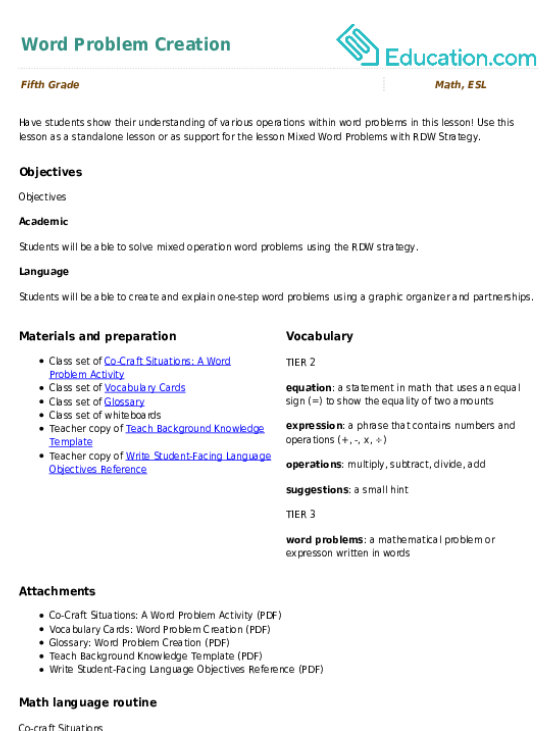Word Problem Creation
Lesson plan
Word Problem Creation
Have students show their understanding of various operations within word problems in this lesson! Use this lesson as a standalone lesson or as support for the lesson Mixed Word Problems with RDW Strategy.
Year 6
Maths
Lesson plan

## Workbooks

No workbooks found for this common core node.

## Games

No games found for this common core node.

## Exercises

No exercises found for this common core node.

### Add to collection

Create new collection

0

### New Collection>

0Items

What could we do to improve Education.com?

Please note: Use the Contact Us link at the bottom of our website for account-specific questions or issues.

What would make you love Education.com?